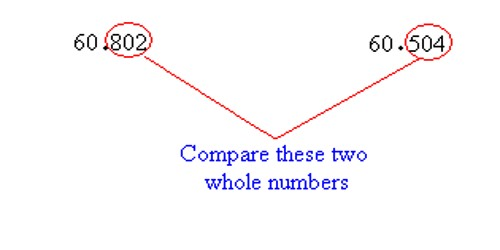Comparing Decimal Numbers

If there are two decimal numbers we can compare them. One number is either greater than, less than or equal to the other number.

A decimal number is just a fractional number. Comparing 0.7 and 0.07 is clearer if we compared 7/10 to 7/100. The fraction 7/10 is equivalent to 70/100 which is clearly larger than 7/100.

Therefore, when decimals are compared start with tenths place and then hundredths place, etc. If one decimal has a higher number in the tenths place then it is larger than a decimal with fewer tenths. If the tenths are equal compare the hundredths, then the thousandths etc. until one decimal is larger or there are no more places to compare. If each decimal place value is the same then the decimals are equal.

Explanation:

The process of comparing decimal numbers is actually exactly the same process for comparing whole numbers, although students might not appreciate this to be the case. Instead of focusing on place value, students might be distracted by the ‘length’ of the whole numbers, and hence base their perception of the relative size of whole numbers on the relative number of digits involved. For example, they might say that “1576 is larger than 742 as it is contains more digits” or “1576 is bigger than 742 as it is longer”. While this strategy will provide correct answers for whole numbers, this lack of focus on place value is likely to lead to similar (but incorrect) strategies for comparing decimal numbers. For example, some students think that long decimals are usually large numbers, and surprisingly, some think that long decimals are usually small numbers.

First, compare the whole numbers to the left of the decimal point.

If they are not the same, the smaller decimal number is the one with the smaller whole number.

For instance, compare 52.432 with 45.989

52 is bigger than 45, so the bigger decimal number is 52.432

We write 52.432 > 45.989 or 45.989 < 52.989

On the other hand, if they are the same, compare the whole number to the right of the decimal point.

The smaller decimal number is the one with the smaller whole number on the right of the decimal point, for instance, compare 60.802 with 60.504The whole numbers to the left of the decimal point are equal, so compare the whole numbers to the right of the decimal point.

504 is smaller than 802, so the smaller decimal number is 60.504.

We write 60.504 < 60.802 or 60.802 > 60.504

Sometimes, they may not have the same number of decimal places to the right of the decimal point.

Just add zero(s) in this case!

For instance, compare 10.598 with 10.61

add a 0 after 61 to get 10.610

610 is bigger than 598, so 10.598 < 10.61

Informational Source: